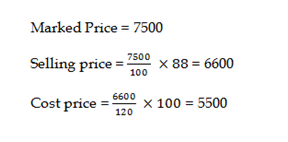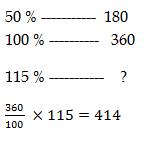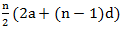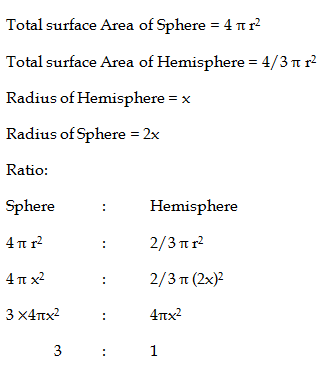# SSC CGL 2018 Practice Test Papers | Quantitative Aptitude (Day-63)

Dear Aspirants, Here we have given the Important SSC CGL Exam 2018 Practice Test Papers. Candidates those who are preparing for SSC CGL 2018 can practice these questions to get more confidence to Crack SSC CGL 2018 Examination.

[WpProQuiz 3818]

Click “Start Quiz” to attend these Questions and view Explanation

1) What is the simplified value of (2 + 1) (22 + 1) (24+ 1) (28 + 1)?

a) 26 – 1

b) 28 – 1

c) 216 – 1

d) 24 – 1

2) What is the smallest value that must be added to 801, so that the resultant is a perfect square?

a) 9

b) 99

c) 40

d) 45

3) If 34N is divisible by 11, then what is the value of N?

a) 0

b) 1

c) 2

d) 3

4) A is four times as efficient as B, A can complete a work in 45 days less than B. If both of them work together, then in how many days the work will be complete?

a) 15 days

b) 10 days

c) 12 days

d) 8 days

5) What will be the net discount (in percentage) after two successive discounts of 20% and 20%?

a) 36

b) 42

c) 64

d) 48

6) In a match, average of runs scored by 5 players is 39. If the runs scored by four players are 55, 30, 42 and 21 respectively, then how many runs did the 5th player had scored?

a) 37

b) 47

c) 67

d) 57

7)  A man gains 20% profit on selling his goods after giving a discount of 12% on marked price. If the marked price is Rs. 7500, then what is the cost price?

a) 6000

b) 6500

c) 5000

d) 5500

8) If 50% of a number is 180, then what is the number which is 15% more than the initial number?

a) 400

b) 450

c) 414

d) 345

9)  A Train starts running with the initial speed of 33 km/hr and its speed increases every hour by a certain amount. If it takes 7 hours to cover a distance of 315 km, then what will be the hourly increment (in km/hr) of the speed of the bus?

a) 6

b) 7

c) 2

d) 4

10) Radius of hemisphere is twice that of a sphere. What is the ratio of total surface area of hemisphere and sphere?

a) 3: 1

b) 4: 1

c) 2: 1

d) 2: 3

(2 + 1) (22 + 1) (24+ 1) (28 + 1)

Using this formula

x2 – 1 => (x+1) (x – 1)

= (22 – 20) (22+22) (24+20) (28+20)
= (24 – 20) (24+20) (28+20)
=(28 – 1) (28+1)
= 216 – 1

801 + 40 = 841

√841 = 29

According the divisibility rule of 11, Difference of Sum of alternative numbers is 0 or 11.

3 + N – 4 = 0

N -1 = 0

N = 1

A         :           B

Efficient         4x        :           1x

Time               1x        :           4x

3x      ———— 45

x         ———–  15

A = 15 days

B = 60 days

A + B = 1/ 15 + 1/ 60 = 5 / 60 = 1/ 12

A and B can complete the work in 12 days

Let cost price = 100

1st discount of 20 % = 100 – 20 = 80 %

2nd Discount of 20 % = 80 – 16 = 64 %

100 – 64 = 36 %

Total scores by 5 players = 39 * 5 = 195

Total score by 4 players = 55 + 30 + 42 + 21 = 148

Score of 5th player = 195 – 148 = 47Initial Speed = 33 kmph

Total Distance = 315 km

Speed of train increase certain amount by every hour is a Arithmetic Progress

AP =Here N = 7, AP = 315

315 = 7 / 2[(2(33) + (7 – 1) d]

315 *2/7 = 66 + 6d

90 = 66 + 6d

24 = 6d

D = 4

Speed of train increases every hour by 4 kmph